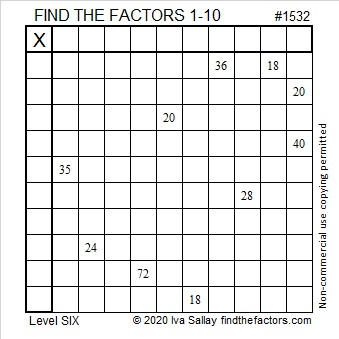# 1532 Don’t Let This Puzzle Spook You!

Contents

### Today’s Puzzle:

If this little ghost prowls your neighborhood this Halloween, don’t let it spook you. Sure, it is a level 6 puzzle, but if you stick to using logic from start to finish, you’ll know the most about this ghost!Here’s the same puzzle minus the embellishments:If you know what a normal distribution is, then you should enjoy this statistics joke:

### Factors of 1532:

• 1532 is a composite number.
• Prime factorization: 1532 = 2 × 2 × 383, which can be written 1532 = 2² × 383
• 1532 has at least one exponent greater than 1 in its prime factorization so √1532 can be simplified. Taking the factor pair from the factor pair table below with the largest square number factor, we get √1532 = (√4)(√383) = 2√383
• The exponents in the prime factorization are 2 and 1. Adding one to each exponent and multiplying we get (2 + 1)(1 + 1) = 3 × 2 = 6. Therefore 1532 has exactly 6 factors.
• The factors of 1532 are outlined with their factor pair partners in the graphic below.### More about the Number 1532:

1532 = 2 × 383 × 2. That factorization looks the same frontwards or backward.

1532 can be written as the difference of two squares:
384² – 382² = 1532.

This site uses Akismet to reduce spam. Learn how your comment data is processed.